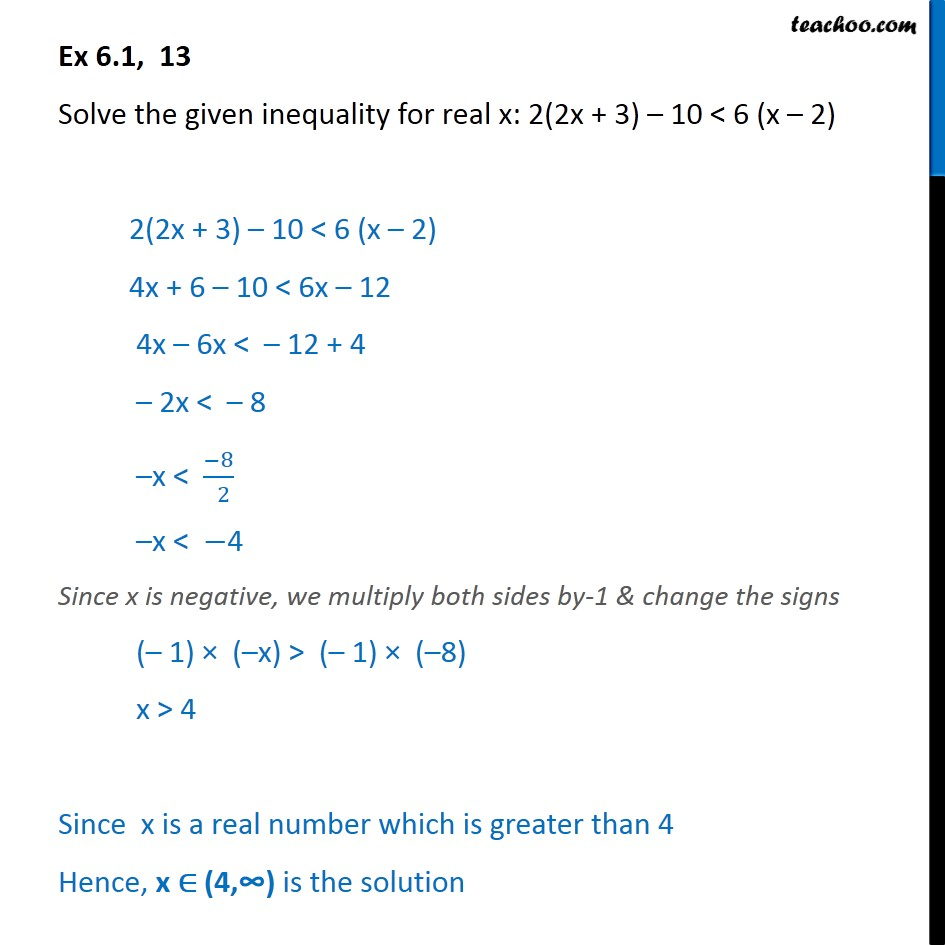Ex 6.1

Chapter 6 Class 11 Linear Inequalities (Term 2)
Serial order wise### Transcript

Ex 6.1, 13 Solve the given inequality for real x: 2(2x + 3) 10 < 6 (x 2) 2(2x + 3) 10 < 6 (x 2) 4x + 6 10 < 6x 12 4x 6x < 12 + 4 2x < 8 x < 8 2 x < 4 Since x is negative, we multiply both sides by-1 & change the signs ( 1) ( x) > ( 1) ( 8) x > 4 Since x is a real number which is greater than 4 Hence, x (4, is the solution